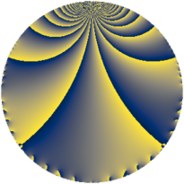# Properties

 Label 7728.2.a.blLevel $7728$ Weight $2$ Character orbit 7728.a Self dual yes Analytic conductor $61.708$ Analytic rank $1$ Dimension $2$ CM no Inner twists $1$

# Related objects

## Newspace parameters

 Level: $$N$$ $$=$$ $$7728 = 2^{4} \cdot 3 \cdot 7 \cdot 23$$ Weight: $$k$$ $$=$$ $$2$$ Character orbit: $$[\chi]$$ $$=$$ 7728.a (trivial)

## Newform invariants

 Self dual: yes Analytic conductor: $$61.7083906820$$ Analytic rank: $$1$$ Dimension: $$2$$ Coefficient field: $$\Q(\sqrt{29})$$ Defining polynomial: $$x^{2} - x - 7$$ Coefficient ring: $$\Z[a_1, \ldots, a_{5}]$$ Coefficient ring index: $$1$$ Twist minimal: no (minimal twist has level 3864) Fricke sign: $$1$$ Sato-Tate group: $\mathrm{SU}(2)$

## $q$-expansion

Coefficients of the $$q$$-expansion are expressed in terms of $$\beta = \frac{1}{2}(1 + \sqrt{29})$$. We also show the integral $$q$$-expansion of the trace form.

 $$f(q)$$ $$=$$ $$q + q^{3} + \beta q^{5} + q^{7} + q^{9} +O(q^{10})$$ $$q + q^{3} + \beta q^{5} + q^{7} + q^{9} -5 q^{11} + ( 1 - \beta ) q^{13} + \beta q^{15} + q^{17} + ( -1 - 2 \beta ) q^{19} + q^{21} - q^{23} + ( 2 + \beta ) q^{25} + q^{27} -5 q^{29} + ( -1 - 2 \beta ) q^{31} -5 q^{33} + \beta q^{35} + ( 1 - 2 \beta ) q^{37} + ( 1 - \beta ) q^{39} - q^{41} + ( -3 + \beta ) q^{43} + \beta q^{45} + ( -6 + 2 \beta ) q^{47} + q^{49} + q^{51} + ( -4 + 3 \beta ) q^{53} -5 \beta q^{55} + ( -1 - 2 \beta ) q^{57} + ( 6 - 3 \beta ) q^{59} + ( 7 - \beta ) q^{61} + q^{63} -7 q^{65} + \beta q^{67} - q^{69} + ( -7 + 3 \beta ) q^{71} -9 q^{73} + ( 2 + \beta ) q^{75} -5 q^{77} + ( 1 - 4 \beta ) q^{79} + q^{81} + ( 7 - 2 \beta ) q^{83} + \beta q^{85} -5 q^{87} + ( -3 - 3 \beta ) q^{89} + ( 1 - \beta ) q^{91} + ( -1 - 2 \beta ) q^{93} + ( -14 - 3 \beta ) q^{95} + ( -9 + 4 \beta ) q^{97} -5 q^{99} +O(q^{100})$$ $$\operatorname{Tr}(f)(q)$$ $$=$$ $$2q + 2q^{3} + q^{5} + 2q^{7} + 2q^{9} + O(q^{10})$$ $$2q + 2q^{3} + q^{5} + 2q^{7} + 2q^{9} - 10q^{11} + q^{13} + q^{15} + 2q^{17} - 4q^{19} + 2q^{21} - 2q^{23} + 5q^{25} + 2q^{27} - 10q^{29} - 4q^{31} - 10q^{33} + q^{35} + q^{39} - 2q^{41} - 5q^{43} + q^{45} - 10q^{47} + 2q^{49} + 2q^{51} - 5q^{53} - 5q^{55} - 4q^{57} + 9q^{59} + 13q^{61} + 2q^{63} - 14q^{65} + q^{67} - 2q^{69} - 11q^{71} - 18q^{73} + 5q^{75} - 10q^{77} - 2q^{79} + 2q^{81} + 12q^{83} + q^{85} - 10q^{87} - 9q^{89} + q^{91} - 4q^{93} - 31q^{95} - 14q^{97} - 10q^{99} + O(q^{100})$$

## Embeddings

For each embedding $$\iota_m$$ of the coefficient field, the values $$\iota_m(a_n)$$ are shown below.

For more information on an embedded modular form you can click on its label.

Label $$\iota_m(\nu)$$ $$a_{2}$$ $$a_{3}$$ $$a_{4}$$ $$a_{5}$$ $$a_{6}$$ $$a_{7}$$ $$a_{8}$$ $$a_{9}$$ $$a_{10}$$
1.1
 −2.19258 3.19258
0 1.00000 0 −2.19258 0 1.00000 0 1.00000 0
1.2 0 1.00000 0 3.19258 0 1.00000 0 1.00000 0
 $$n$$: e.g. 2-40 or 990-1000 Significant digits: Format: Complex embeddings Normalized embeddings Satake parameters Satake angles

## Atkin-Lehner signs

$$p$$ Sign
$$2$$ $$1$$
$$3$$ $$-1$$
$$7$$ $$-1$$
$$23$$ $$1$$

## Inner twists

This newform does not admit any (nontrivial) inner twists.

## Twists

By twisting character orbit
Char Parity Ord Mult Type Twist Min Dim
1.a even 1 1 trivial 7728.2.a.bl 2
4.b odd 2 1 3864.2.a.h 2

By twisted newform orbit
Twist Min Dim Char Parity Ord Mult Type
3864.2.a.h 2 4.b odd 2 1
7728.2.a.bl 2 1.a even 1 1 trivial

## Hecke kernels

This newform subspace can be constructed as the intersection of the kernels of the following linear operators acting on $$S_{2}^{\mathrm{new}}(\Gamma_0(7728))$$:

 $$T_{5}^{2} - T_{5} - 7$$ $$T_{11} + 5$$ $$T_{13}^{2} - T_{13} - 7$$ $$T_{17} - 1$$

## Hecke characteristic polynomials

$p$ $F_p(T)$
$2$ $$T^{2}$$
$3$ $$( -1 + T )^{2}$$
$5$ $$-7 - T + T^{2}$$
$7$ $$( -1 + T )^{2}$$
$11$ $$( 5 + T )^{2}$$
$13$ $$-7 - T + T^{2}$$
$17$ $$( -1 + T )^{2}$$
$19$ $$-25 + 4 T + T^{2}$$
$23$ $$( 1 + T )^{2}$$
$29$ $$( 5 + T )^{2}$$
$31$ $$-25 + 4 T + T^{2}$$
$37$ $$-29 + T^{2}$$
$41$ $$( 1 + T )^{2}$$
$43$ $$-1 + 5 T + T^{2}$$
$47$ $$-4 + 10 T + T^{2}$$
$53$ $$-59 + 5 T + T^{2}$$
$59$ $$-45 - 9 T + T^{2}$$
$61$ $$35 - 13 T + T^{2}$$
$67$ $$-7 - T + T^{2}$$
$71$ $$-35 + 11 T + T^{2}$$
$73$ $$( 9 + T )^{2}$$
$79$ $$-115 + 2 T + T^{2}$$
$83$ $$7 - 12 T + T^{2}$$
$89$ $$-45 + 9 T + T^{2}$$
$97$ $$-67 + 14 T + T^{2}$$